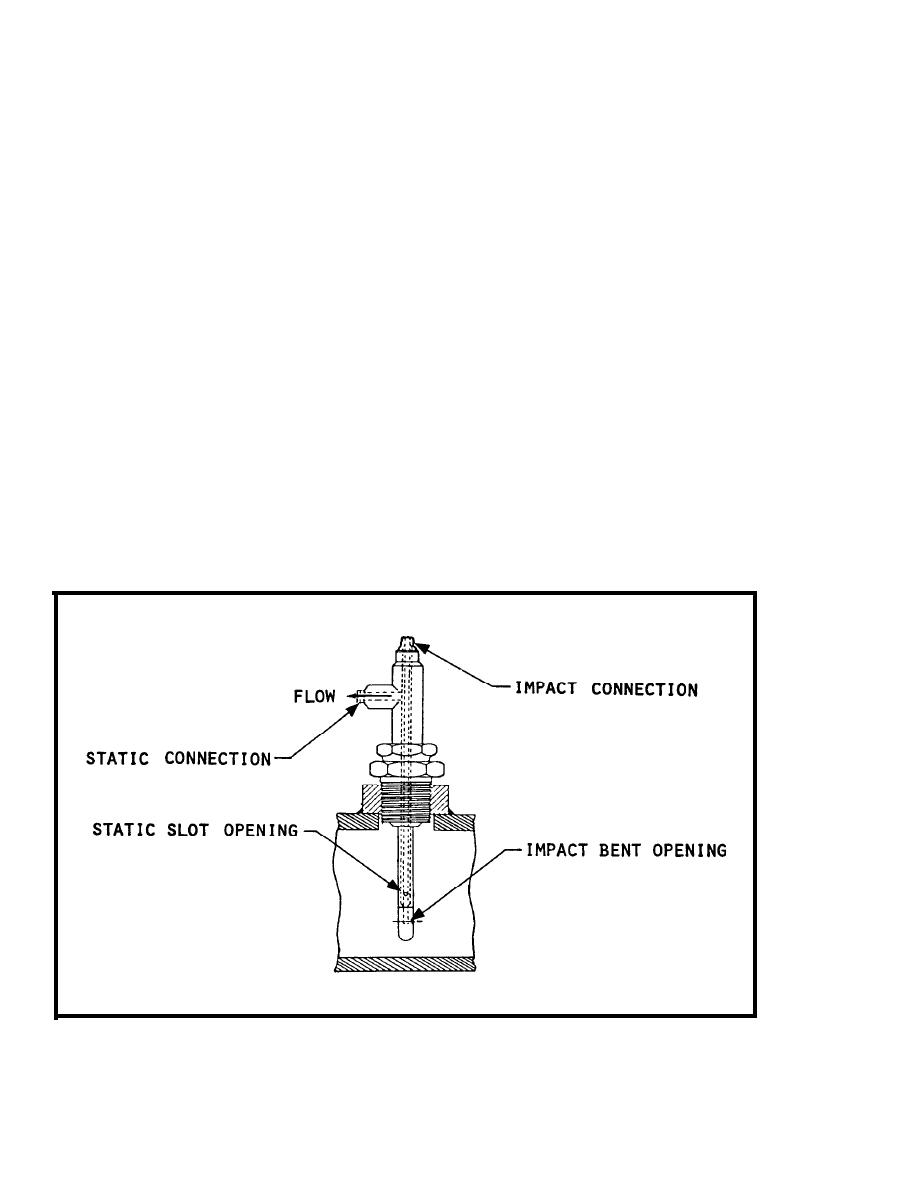Figure 3-15. Pitot Tubes Mounted in a Single CasingCustom Search( f ) I n s t a l l a t i o n .  Shutdown of the steam system at installation, and
e a c h time the orifice plate is checked or replaced, is necessary. An upstream
s t r a i g h t pipe run of 10 to 30 diameters is required.
3 . 2 . 2 P i t o t T u b e s .  T h e pitot tube, which is limited to single point
measurements, is another type of DP meter.  Pitot tubes sense two pressures at
the same time, i m p a c t and static.  An impact tube is open ended and has a 90
b e n d at the bottom; a static tube is straight, closed at the bottom, with a
s l o t in the side.  The tubes may be mounted separately or combined coaxially
as shown in figure 3-15.  Pressure taps are connected to a manometer where the
p r e s s u r e d i f f e r e n t i a l i s i n d i c a t e d .  I t is common installation practice to
w e l d a coupling to a pipe and insert the probe through the coupling.
Pi tot
tubes have a minimum pressure drop and are low in cost, free of moving parts,
a n d easy to install, but are susceptible to being plugged by suspended matter
in the fluid.
3 . 2 . 3 Insertion Turbine Meters.
F i x e d and insertion turbine meters (figures
3 - 1 1 and 3-12) measure velocity of steam in a pipe with a rotor inserted at
t h e c r i t i c a l p o i n t i n t h e f l o w p r o f i l e .  T h e critical point represents the
a v e r a g e flow velocity.  T h e following items are characteristics of insertion
t u r b i n e meters.
( a ) Accuracy.  A c c u r a c y within the turndown ratio of 25:1 is typically
%1 percent.  T h e t u r n d o w n r a t i o i s s i g n i f i c a n t l y b e t t e r t h a n d i f f e r e n t i a l
pressure meters.
( b ) S i z i n g .  Sizing is less critical than differential pressure meters
b e c a u s e of wide rotor range and ease of installation.
FIGURE 3-15.
Pitot Tubes Mounted in a Single Casing
3-48Integrated Publishing, Inc.